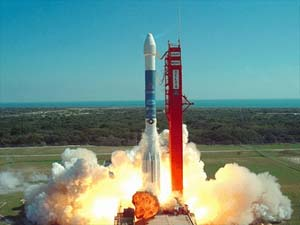# Watch that satellite being launched!Calculate the work that is done in Joules in launching a satellite of mass 1000 kg into an orbit above the Earth, at an elevation equal to the radius of the Earth?

Details and Assumptions

• Earth’s mass = $6\times 10^{24}$ kg
• Earth’s radius = $6.4 \times 10^6$ m
• You may assume that all the work applied to the satellite is transformed into potential energy.
×

Problem Loading...

Note Loading...

Set Loading...